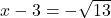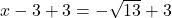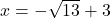## Find the zeros of y = x2 – 6x – 4 by completing the square.

Question

Find the zeros of y = x2 – 6x – 4 by completing the square.

in progress 0
1 month 2021-08-13T20:35:45+00:00 1 Answers 2 views 0

The solutions to the quadratic equations are:Step-by-step explanation:

Given the function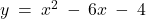substitute y = 0 in the equation to determine the zeros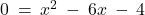Switch sides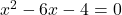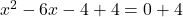Simplify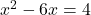Rewrite in the form (x+a)² = b

But, in order to rewrite in the form x²+2ax+a²

Solve for ‘a’

2ax = -6x

a = -3

so add a² = (-3)² to both sides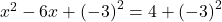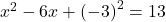Apply perfect square formula:  (a-b)² = a²-2ab+b²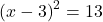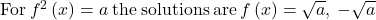solve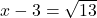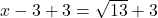Simplify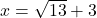now solving